# http www comp nus edu sgcs 1010 UNIT

• Slides: 61http: //www. comp. nus. edu. sg/~cs 1010/ UNIT 6 Problem Solving with Selection and Repetition Statements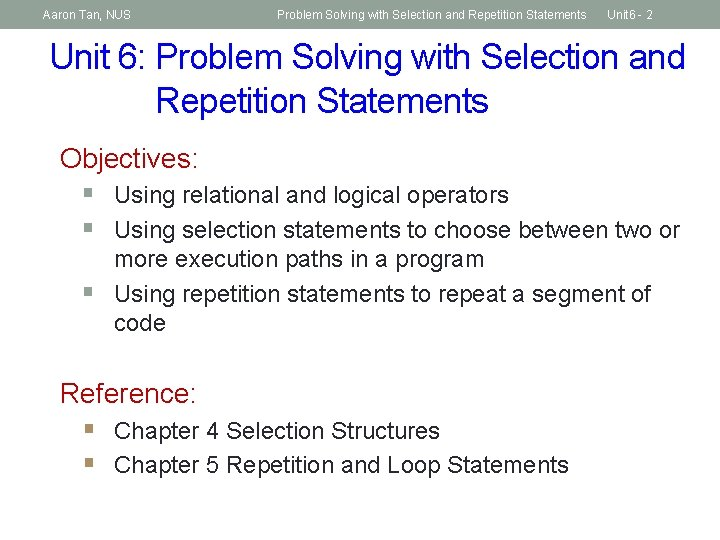Aaron Tan, NUS Problem Solving with Selection and Repetition Statements Unit 6 - 2 Unit 6: Problem Solving with Selection and Repetition Statements Objectives: § Using relational and logical operators § Using selection statements to choose between two or § more execution paths in a program Using repetition statements to repeat a segment of code Reference: § Chapter 4 Selection Structures § Chapter 5 Repetition and Loop StatementsAaron Tan, NUS Problem Solving with Selection and Repetition Statements Unit 6 - 3 Unit 6: Problem Solving with Selection and Repetition Statements (1/2) 1. Sequential vs Non-Sequential Control Flow 2. Selection Structures 3. Nested if and if-else Statements 4. Style Issues 5. Common Errors 6. The switch Statement 7. Testing and Debugging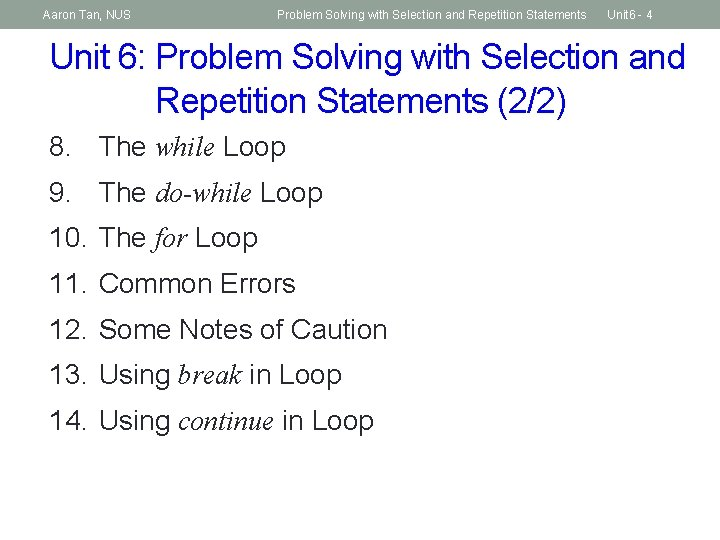Aaron Tan, NUS Problem Solving with Selection and Repetition Statements Unit 6 - 4 Unit 6: Problem Solving with Selection and Repetition Statements (2/2) 8. The while Loop 9. The do-while Loop 10. The for Loop 11. Common Errors 12. Some Notes of Caution 13. Using break in Loop 14. Using continue in LoopAaron Tan, NUS Problem Solving with Selection and Repetition Statements Recall: Control Structures Sequence Selection Repetition Unit 6 - 5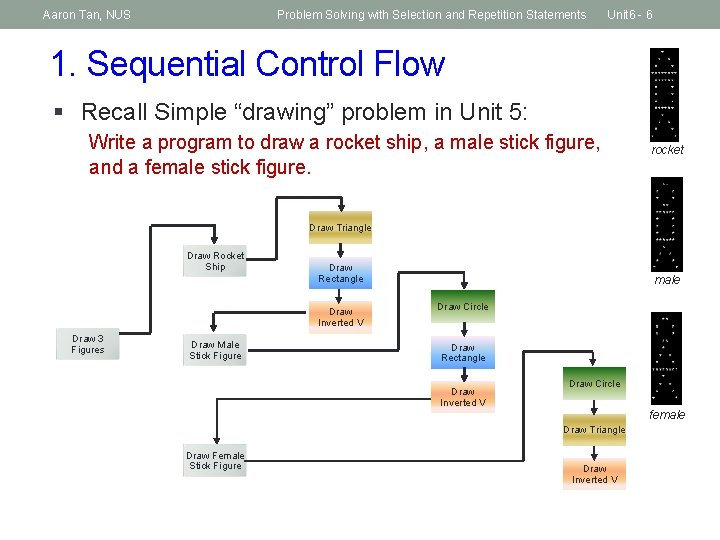Aaron Tan, NUS Problem Solving with Selection and Repetition Statements Unit 6 - 6 1. Sequential Control Flow § Recall Simple “drawing” problem in Unit 5: Write a program to draw a rocket ship, a male stick figure, and a female stick figure. rocket Draw Triangle Draw Rocket Ship Draw Rectangle Draw Inverted V Draw 3 Figures Draw Male Stick Figure male Draw Circle Draw Rectangle Draw Inverted V Draw Circle female Draw Triangle Draw Female Stick Figure Draw Inverted V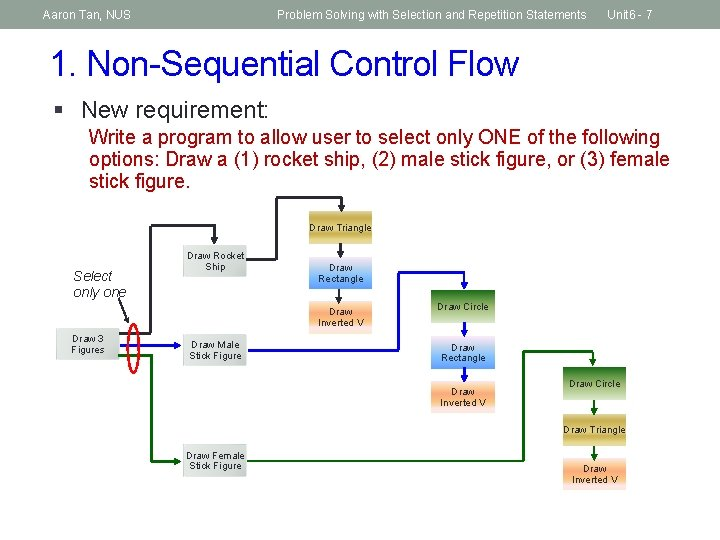Aaron Tan, NUS Problem Solving with Selection and Repetition Statements Unit 6 - 7 1. Non-Sequential Control Flow § New requirement: Write a program to allow user to select only ONE of the following options: Draw a (1) rocket ship, (2) male stick figure, or (3) female stick figure. Draw Triangle Select only one Draw Rocket Ship Draw Rectangle Draw Inverted V Draw 3 Figures Draw Male Stick Figure Draw Circle Draw Rectangle Draw Inverted V Draw Circle Draw Triangle Draw Female Stick Figure Draw Inverted VAaron Tan, NUS Problem Solving with Selection and Repetition Statements Unit 6 - 8 2. Selection Structures § C provides two control structures that allow you to select a group of statements to be executed or skipped when certain conditions are met.Aaron Tan, NUS Problem Solving with Selection and Repetition Statements Unit 6 - 9 2. 1 if and if-else Statements true § if statement cond? How are conditions specified and how are they evaluated? false if ( condition ) { /* Execute these statements if TRUE */ } Braces { } are optional only if there is one statement in the block. But for beginners, we recommended writing braces even if there is one statement. true § if-else statement if ( condition ) { /* Execute these statements if TRUE */ } else { /* Execute these statements if FALSE */ } cond? false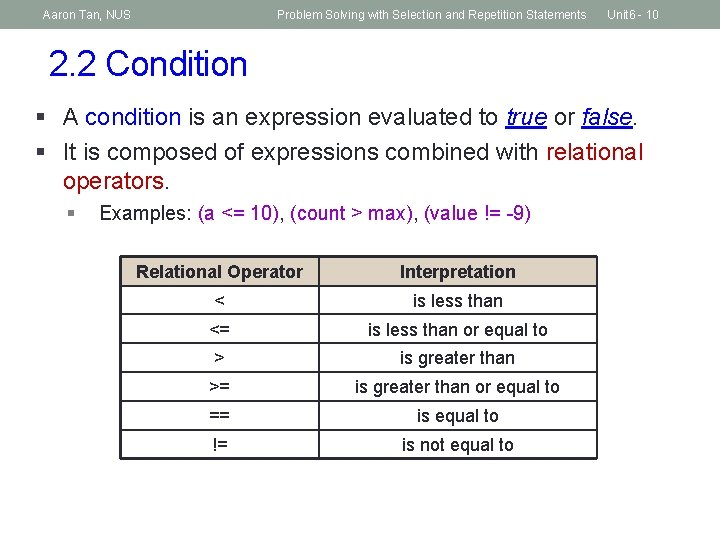Aaron Tan, NUS Problem Solving with Selection and Repetition Statements Unit 6 - 10 2. 2 Condition § A condition is an expression evaluated to true or false. § It is composed of expressions combined with relational operators. § Examples: (a <= 10), (count > max), (value != -9) Relational Operator Interpretation < is less than <= is less than or equal to > is greater than >= is greater than or equal to == is equal to != is not equal toAaron Tan, NUS Problem Solving with Selection and Repetition Statements Unit 6 - 11 2. 3 Truth Values § Boolean values: true or false. § There is no boolean type in ANSI C. Instead, we use integers: § § 0 to represent false Any other value to represent true (1 is used as the representative value for true in output) § Example: int a = (2 > 3); int b = (3 > 2); Unit 6_Truth. Values. c printf("a = %d; b = %dn", a, b); a = 0; b = 1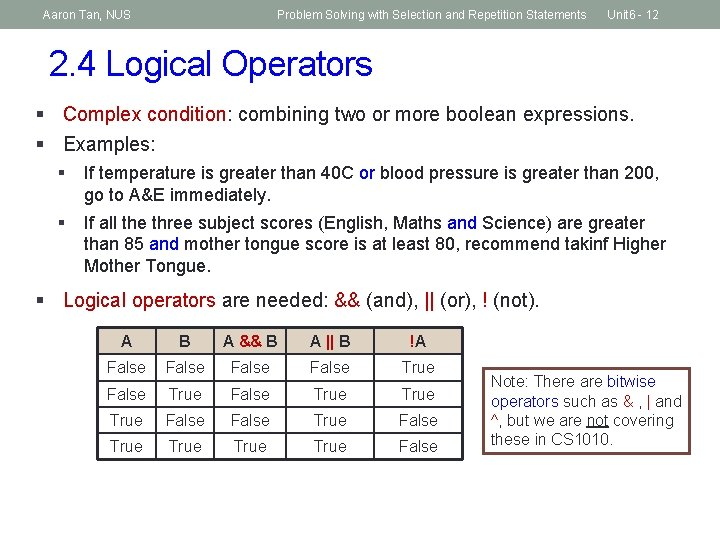Aaron Tan, NUS Problem Solving with Selection and Repetition Statements Unit 6 - 12 2. 4 Logical Operators § Complex condition: combining two or more boolean expressions. § Examples: § If temperature is greater than 40 C or blood pressure is greater than 200, go to A&E immediately. § If all the three subject scores (English, Maths and Science) are greater than 85 and mother tongue score is at least 80, recommend takinf Higher Mother Tongue. § Logical operators are needed: && (and), || (or), ! (not). A B A && B A || B !A False True False True True False Note: There are bitwise operators such as & , | and ^, but we are not covering these in CS 1010.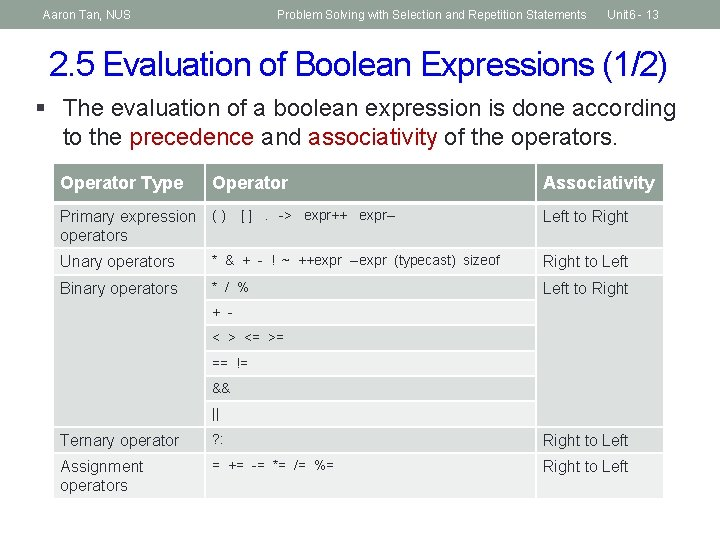Aaron Tan, NUS Problem Solving with Selection and Repetition Statements Unit 6 - 13 2. 5 Evaluation of Boolean Expressions (1/2) § The evaluation of a boolean expression is done according to the precedence and associativity of the operators. Operator Type Operator Primary expression ( ) [ ]. -> expr++ expr-operators Associativity Left to Right Unary operators * & + - ! ~ ++expr --expr (typecast) sizeof Right to Left Binary operators * / % Left to Right + < > <= >= == != && || Ternary operator ? : Right to Left Assignment operators = += -= *= /= %= Right to Left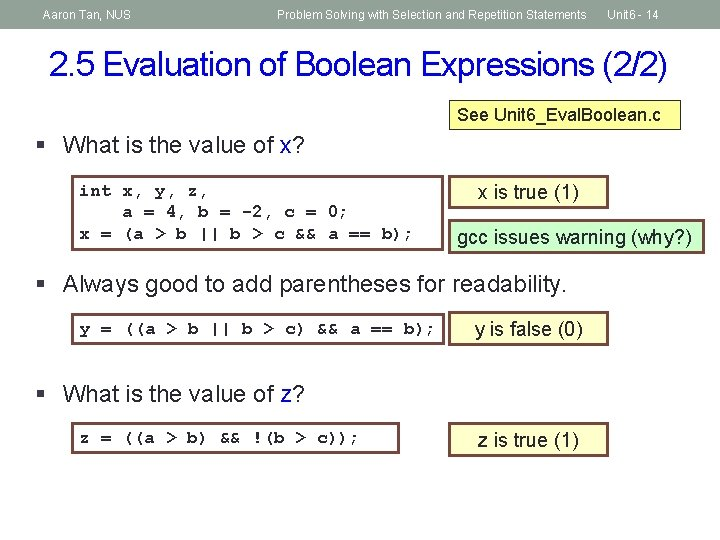Aaron Tan, NUS Problem Solving with Selection and Repetition Statements Unit 6 - 14 2. 5 Evaluation of Boolean Expressions (2/2) See Unit 6_Eval. Boolean. c § What is the value of x? int x, y, z, a = 4, b = -2, c = 0; x = (a > b || b > c && a == b); x is true (1) gcc issues warning (why? ) § Always good to add parentheses for readability. y = ((a > b || b > c) && a == b); y is false (0) § What is the value of z? z = ((a > b) && !(b > c)); z is true (1)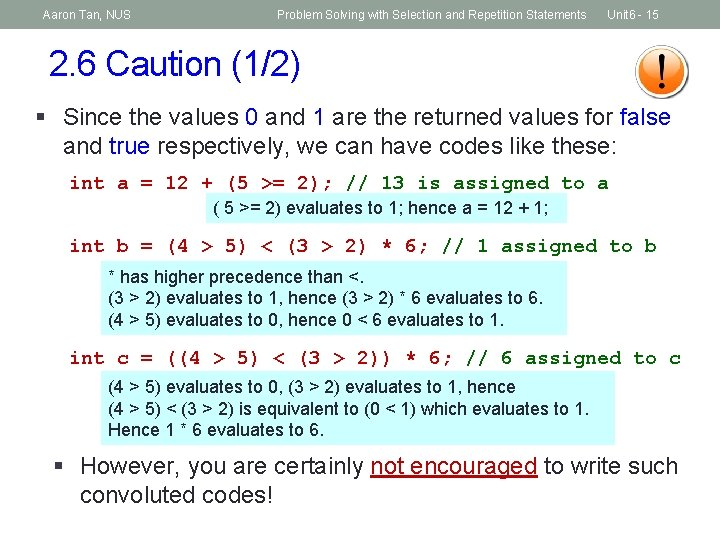Aaron Tan, NUS Problem Solving with Selection and Repetition Statements Unit 6 - 15 2. 6 Caution (1/2) § Since the values 0 and 1 are the returned values for false and true respectively, we can have codes like these: int a = 12 + (5 >= 2); // 13 is assigned to a ( 5 >= 2) evaluates to 1; hence a = 12 + 1; int b = (4 > 5) < (3 > 2) * 6; // 1 assigned to b * has higher precedence than <. (3 > 2) evaluates to 1, hence (3 > 2) * 6 evaluates to 6. (4 > 5) evaluates to 0, hence 0 < 6 evaluates to 1. int c = ((4 > 5) < (3 > 2)) * 6; // 6 assigned to c (4 > 5) evaluates to 0, (3 > 2) evaluates to 1, hence (4 > 5) < (3 > 2) is equivalent to (0 < 1) which evaluates to 1. Hence 1 * 6 evaluates to 6. § However, you are certainly not encouraged to write such convoluted codes!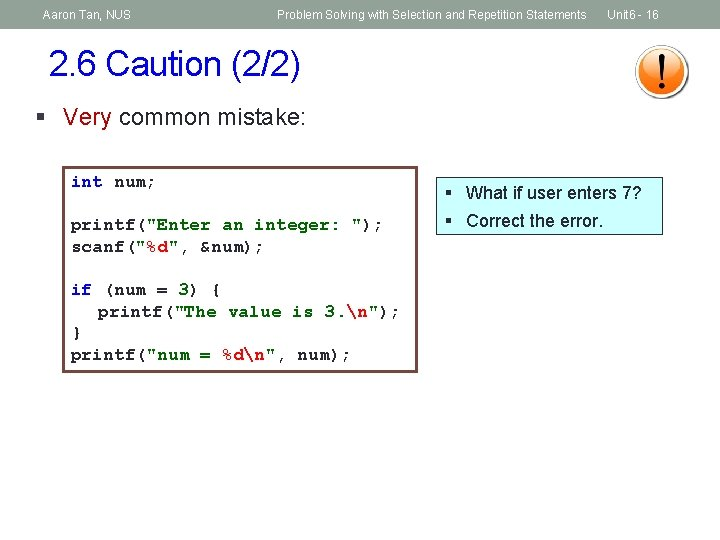Aaron Tan, NUS Problem Solving with Selection and Repetition Statements Unit 6 - 16 2. 6 Caution (2/2) § Very common mistake: int num; printf("Enter an integer: "); scanf("%d", &num); if (num = 3) { printf("The value is 3. n"); } printf("num = %dn", num); § What if user enters 7? § Correct the error.Aaron Tan, NUS Problem Solving with Selection and Repetition Statements Unit 6 - 17 2. 7 Short-Circuit Evaluation § Does the following code give an error if variable a is zero? if ((a != 0) && (b/a > 3)) printf(. . . ); § Short-circuit evaluation § expr 1 || expr 2: If expr 1 is true, skip evaluating expr 2 and return true immediately, as the result will always be true. § expr 1 && expr 2: If expr 1 is false, skip evaluating expr 2 and return false immediately, as the result will always be false.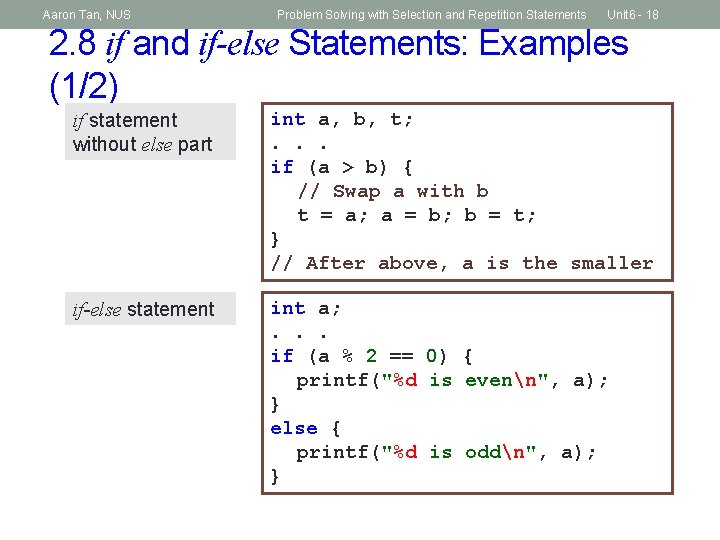Aaron Tan, NUS Problem Solving with Selection and Repetition Statements Unit 6 - 18 2. 8 if and if-else Statements: Examples (1/2) if statement without else part int a, b, t; . . . if (a > b) { // Swap a with b t = a; a = b; b = t; } // After above, a is the smaller if-else statement int a; . . . if (a % 2 == 0) { printf("%d is evenn", a); } else { printf("%d is oddn", a); }Aaron Tan, NUS Problem Solving with Selection and Repetition Statements Unit 6 - 19 2. 8 if and if-else Statements: Examples (2/2) § Move common statements out of the if-else construct. if (cond) { statement-a; statement-b; statement-j; statement-x; statement-y; } else { statement-a; statement-b; statement-k; statement-x; statement-y; } statement-a; statement-b; if (cond) { statement-j; } else { statement-k; } statement-x; statement-y;Aaron Tan, NUS Problem Solving with Selection and Repetition Statements Unit 6 - 20 3. Nested if and if-else Statements (1/2) § Nested if (if-else) structures refer to the containment of an if (if-else) structure within another if (if-else) structure. § For example: § If it is a weekday, you will be in school from 8 am to 6 pm, do revision from 6 pm to 12 midnight, and sleep from 12 midnight to 8 am. § If it is a weekend, then you will sleep from 12 midnight to 10 am and have fun from 10 am to 12 midnight.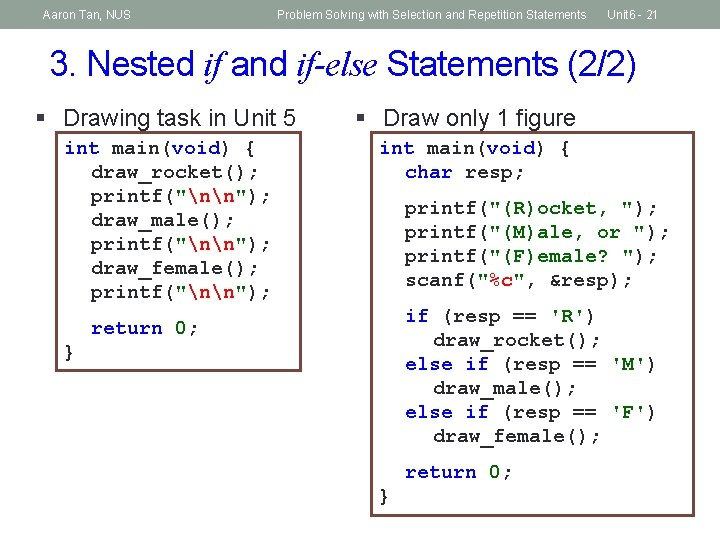Aaron Tan, NUS Problem Solving with Selection and Repetition Statements Unit 6 - 21 3. Nested if and if-else Statements (2/2) § Drawing task in Unit 5 int main(void) { draw_rocket(); printf("nn"); draw_male(); printf("nn"); draw_female(); printf("nn"); § Draw only 1 figure int main(void) { char resp; printf("(R)ocket, "); printf("(M)ale, or "); printf("(F)emale? "); scanf("%c", &resp); if (resp == 'R') draw_rocket(); else if (resp == 'M') draw_male(); else if (resp == 'F') draw_female(); return 0; }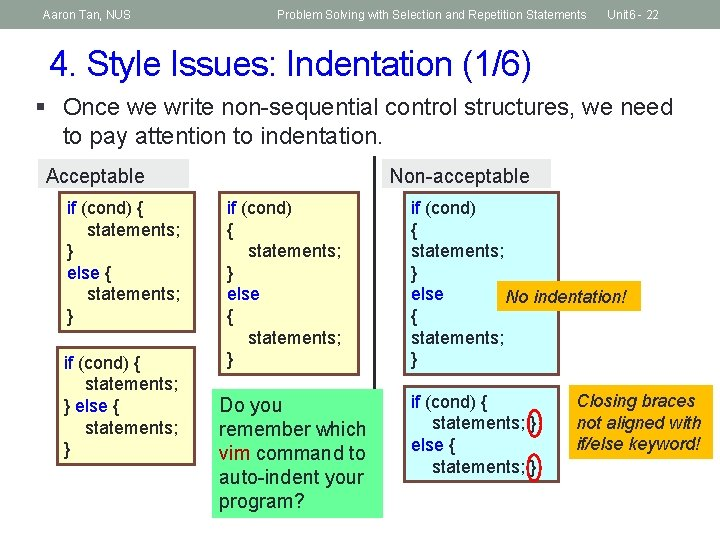Aaron Tan, NUS Problem Solving with Selection and Repetition Statements Unit 6 - 22 4. Style Issues: Indentation (1/6) § Once we write non-sequential control structures, we need to pay attention to indentation. Acceptable if (cond) { statements; } else { statements; } Non-acceptable if (cond) { statements; } else { statements; } Do you remember which vim command to auto-indent your program? if (cond) { statements; } else No indentation! { statements; } if (cond) { statements; } else { statements; } Closing braces not aligned with if/else keyword!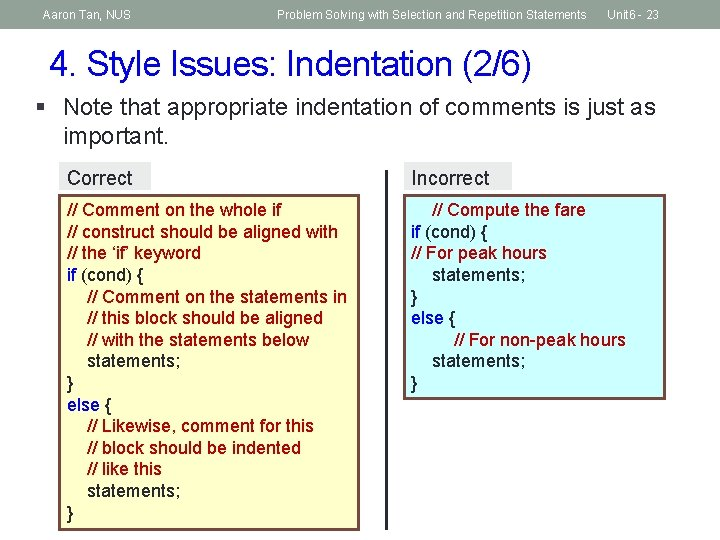Aaron Tan, NUS Problem Solving with Selection and Repetition Statements Unit 6 - 23 4. Style Issues: Indentation (2/6) § Note that appropriate indentation of comments is just as important. Correct Incorrect // Comment on the whole if // construct should be aligned with // the ‘if’ keyword if (cond) { // Comment on the statements in // this block should be aligned // with the statements below statements; } else { // Likewise, comment for this // block should be indented // like this statements; } // Compute the fare if (cond) { // For peak hours statements; } else { // For non-peak hours statements; }Aaron Tan, NUS Problem Solving with Selection and Repetition Statements Unit 6 - 24 4. Style Issues: Indentation (3/6) § Sometimes we may have a deeply nested if-else-if construct: int marks; char grade; . . . if (marks >= 90) grade = 'A'; else if (marks >= 75) grade = 'B'; else if (marks >= 60) grade = 'C'; else if (marks >= 50) grade = 'D'; else grade = 'F'; § This follows the indentation guideline, but in this case the code tends to be long and it skews too much to the right.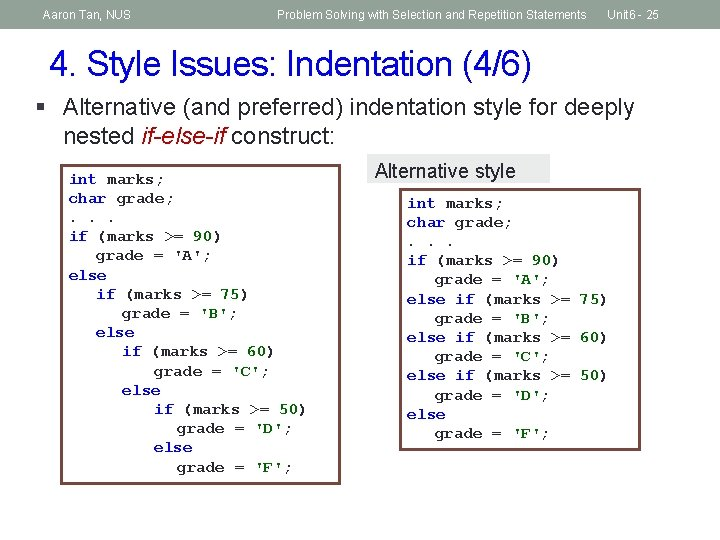Aaron Tan, NUS Problem Solving with Selection and Repetition Statements Unit 6 - 26 4. Style Issues: Naming ‘boolean’ variables (5/6) § Here, ‘boolean’ variables refer to int variables which are used to hold 1 or 0 to represent true or false respectively. § These are also known as boolean flags. § To improve readability, boolean flags should be given descriptive names just like any other variables. § In general, add suffices such as “is” or “has” to names of boolean flags (instead of just calling them “flag”!) § Example: is. Even, is. Prime, has. Error, has. Duplicates int is. Even, . . . if (num % 2 is. Even = else is. Even = num; == 0) 1; 0;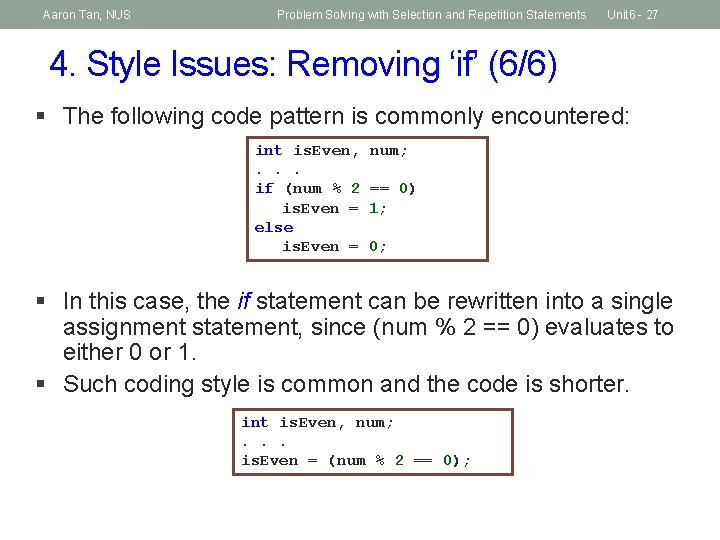Aaron Tan, NUS Problem Solving with Selection and Repetition Statements Unit 6 - 27 4. Style Issues: Removing ‘if’ (6/6) § The following code pattern is commonly encountered: int is. Even, . . . if (num % 2 is. Even = else is. Even = num; == 0) 1; 0; § In this case, the if statement can be rewritten into a single assignment statement, since (num % 2 == 0) evaluates to either 0 or 1. § Such coding style is common and the code is shorter. int is. Even, num; . . . is. Even = (num % 2 == 0);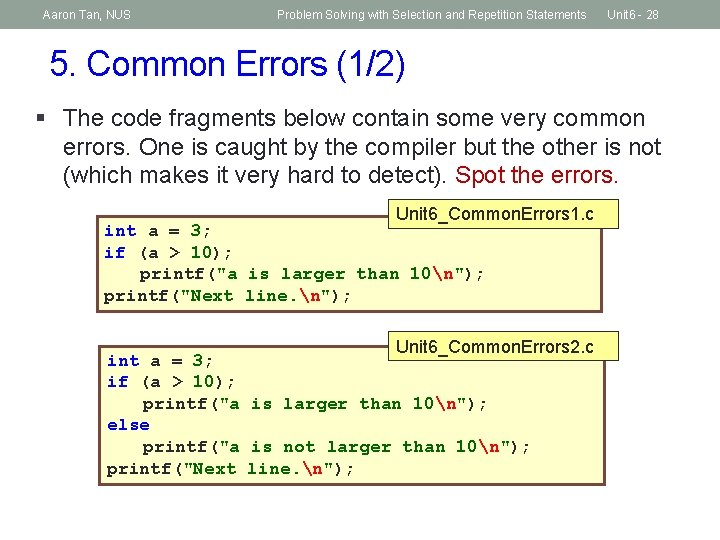Aaron Tan, NUS Problem Solving with Selection and Repetition Statements Unit 6 - 28 5. Common Errors (1/2) § The code fragments below contain some very common errors. One is caught by the compiler but the other is not (which makes it very hard to detect). Spot the errors. Unit 6_Common. Errors 1. c int a = 3; if (a > 10); printf("a is larger than 10n"); printf("Next line. n"); Unit 6_Common. Errors 2. c int a = 3; if (a > 10); printf("a is larger than 10n"); else printf("a is not larger than 10n"); printf("Next line. n");Aaron Tan, NUS Problem Solving with Selection and Repetition Statements Unit 6 - 29 5. Common Errors (2/2) § Proper indentation is important. In the following code, the indentation does not convey the intended purpose of the code. Why? Which if is the else matched to? int a, b; . . . Unit 6_Common. Errors 3. c if (a > 10) if (b < 9) printf("Hellon"); else printf("Goodbyen"); Same as int a, b; . . . if (a > 10) if (b < 9) printf("Hellon"); else printf("Goodbyen"); int a, b; . . . Use braces if you want to make it more readable: if (a > 10) { if (b < 9) printf("Hellon"); else printf("Goodbyen"); }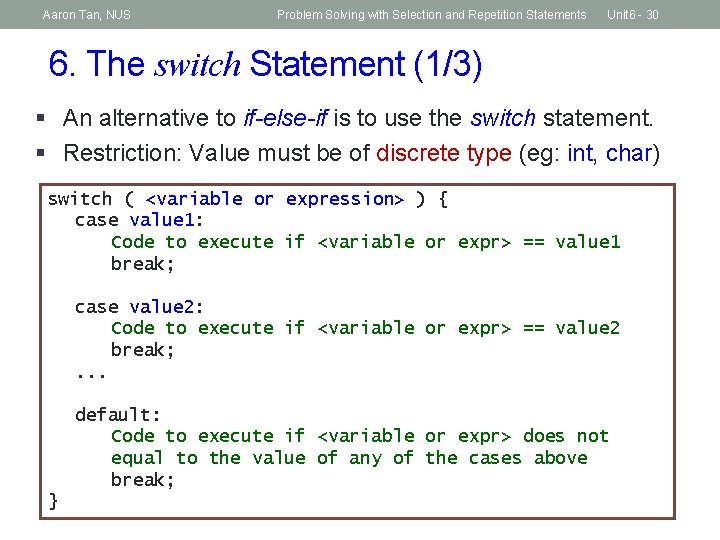Aaron Tan, NUS Problem Solving with Selection and Repetition Statements Unit 6 - 30 6. The switch Statement (1/3) § An alternative to if-else-if is to use the switch statement. § Restriction: Value must be of discrete type (eg: int, char) switch ( <variable or expression> ) { case value 1: Code to execute if <variable or expr> == value 1 break; case value 2: Code to execute if <variable or expr> == value 2 break; . . . default: Code to execute if <variable or expr> does not equal to the value of any of the cases above break; }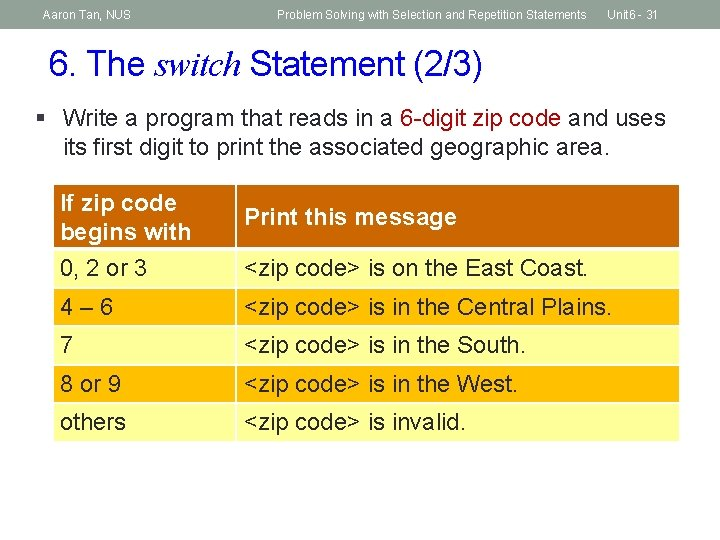Aaron Tan, NUS Problem Solving with Selection and Repetition Statements Unit 6 - 31 6. The switch Statement (2/3) § Write a program that reads in a 6 -digit zip code and uses its first digit to print the associated geographic area. If zip code begins with Print this message 0, 2 or 3 <zip code> is on the East Coast. 4– 6 <zip code> is in the Central Plains. 7 <zip code> is in the South. 8 or 9 <zip code> is in the West. others <zip code> is invalid.Aaron Tan, NUS Problem Solving with Selection and Repetition Statements 6. The switch Statement (3/3) #include <stdio. h> int main(void) { int zip; Unit 6_Zip. Code. c printf("Enter a 6 -digit ZIP code: "); scanf("%d", &zip); switch (zip/100000) { case 0: case 2: case 3: printf("%06 d is on the East Coast. n", zip); break; case 4: case 5: case 6: printf("%d is in the Central Plains. n", zip); break; case 7: printf("%d is in the South. n", zip); break; case 8: case 9: printf("%d is in the West. n", zip); break; default: printf("%d is invalid. n", zip); } // end switch return 0; } Unit 6 - 32Aaron Tan, NUS Problem Solving with Selection and Repetition Statements Unit 6 - 33 7. Testing and Debugging (1/3) § Finding the maximum value among 3 variables: // Returns largest among num 1, num 2, num 3 int get. Max(int num 1, int num 2, int num 3) { int max = 0; if ((num 1 > num 2) && (num 1 > num 3)) max = num 1; if ((num 2 > num 1) && (num 2 > num 3)) max = num 2; if ((num 3 > num 1) && (num 3 > num 2)) max = num 3; return max; } Unit 6_Find. Max_v 1. c § What is wrong with the code? Did you test it with the correct test data? § What test data would expose the flaw of the code? § How do you correct the code? § After correcting the code, would replacing the 3 if statements with a nested if-else statement work? If it works, which method is better?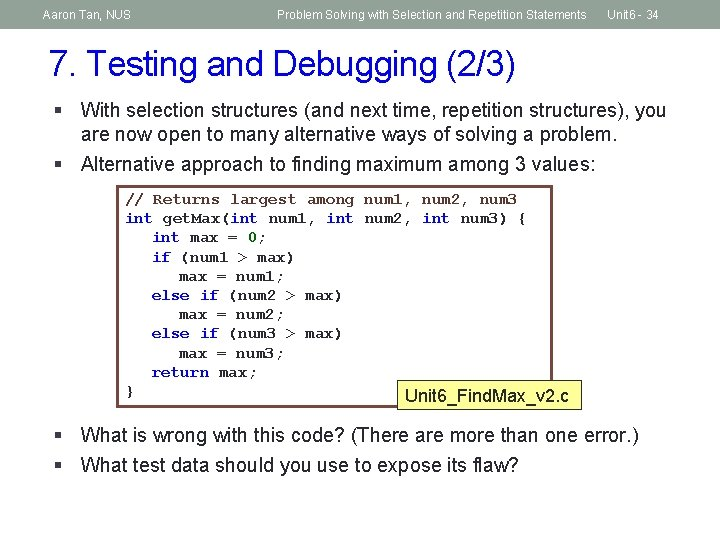Aaron Tan, NUS Problem Solving with Selection and Repetition Statements Unit 6 - 34 7. Testing and Debugging (2/3) § With selection structures (and next time, repetition structures), you are now open to many alternative ways of solving a problem. § Alternative approach to finding maximum among 3 values: // Returns largest among num 1, num 2, num 3 int get. Max(int num 1, int num 2, int num 3) { int max = 0; if (num 1 > max) max = num 1; else if (num 2 > max) max = num 2; else if (num 3 > max) max = num 3; return max; } Unit 6_Find. Max_v 2. c § What is wrong with this code? (There are more than one error. ) § What test data should you use to expose its flaw?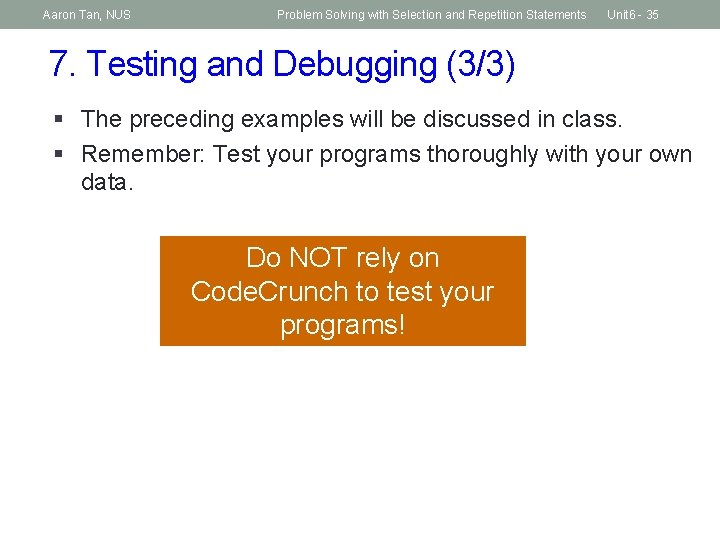Aaron Tan, NUS Problem Solving with Selection and Repetition Statements Unit 6 - 35 7. Testing and Debugging (3/3) § The preceding examples will be discussed in class. § Remember: Test your programs thoroughly with your own data. Do NOT rely on Code. Crunch to test your programs!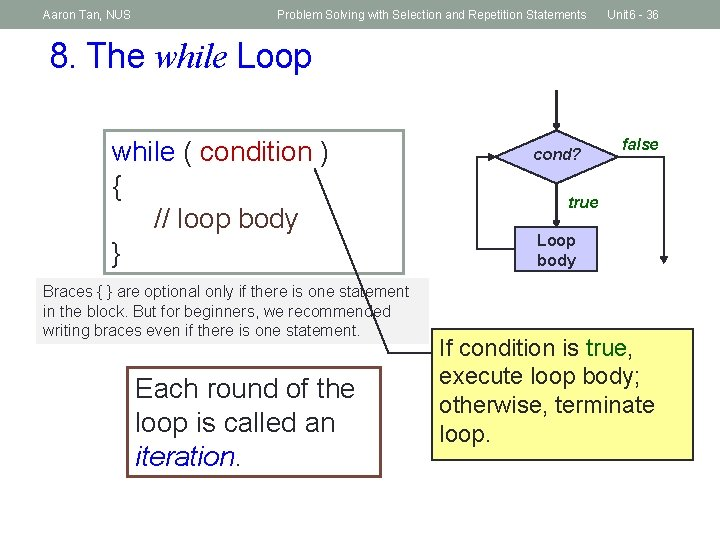Aaron Tan, NUS Problem Solving with Selection and Repetition Statements Unit 6 - 36 8. The while Loop while ( condition ) { // loop body } Braces { } are optional only if there is one statement in the block. But for beginners, we recommended writing braces even if there is one statement. Each round of the loop is called an iteration. cond? false true Loop body If condition is true, execute loop body; otherwise, terminate loop.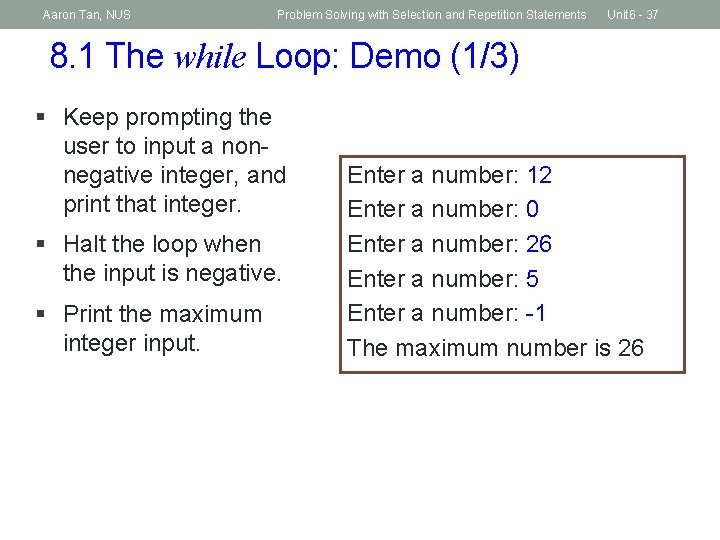Aaron Tan, NUS Problem Solving with Selection and Repetition Statements Unit 6 - 37 8. 1 The while Loop: Demo (1/3) § Keep prompting the user to input a nonnegative integer, and print that integer. § Halt the loop when the input is negative. § Print the maximum integer input. Enter a number: 12 Enter a number: 0 Enter a number: 26 Enter a number: 5 Enter a number: -1 The maximum number is 26Aaron Tan, NUS Problem Solving with Selection and Repetition Statements Unit 6 - 38 8. 1 The while Loop: Demo (2/3) maxi = 0; read num; if (num >= 0) { if (maxi < num) maxi = num; read num; } else stop; . . . print maxi; maxi = 0; read num; while (num >= 0) { if (maxi < num) maxi = num; read num; } print maxi;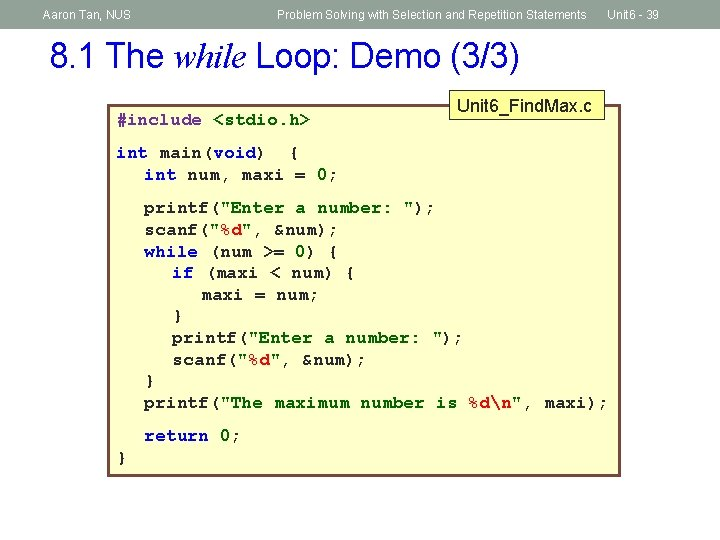Aaron Tan, NUS Problem Solving with Selection and Repetition Statements Unit 6 - 39 8. 1 The while Loop: Demo (3/3) #include <stdio. h> Unit 6_Find. Max. c int main(void) { int num, maxi = 0; printf("Enter a number: "); scanf("%d", &num); while (num >= 0) { if (maxi < num) { maxi = num; } printf("Enter a number: "); scanf("%d", &num); } printf("The maximum number is %dn", maxi); return 0; }Aaron Tan, NUS Problem Solving with Selection and Repetition Statements Unit 6 - 40 8. 2 Condition for while Loop // pseudo-code a = 2; b = 7; while (a == b) { print a; a = a + 2; } // pseudo-code a = 2; b = 7; while (a != b) { print a; a = a + 2; } Output: ? § When the loop condition is always false, the loop body is not executed. Output: ? 2 4 6 8 10 : Press ctrl-c to interrupt § When the loop condition is always true, the loop body is executed forever – infinite loop.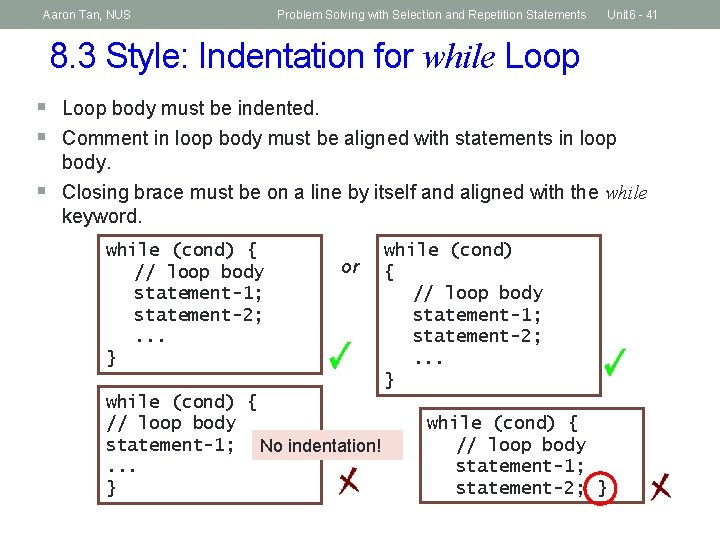Aaron Tan, NUS Problem Solving with Selection and Repetition Statements Unit 6 - 41 8. 3 Style: Indentation for while Loop § Loop body must be indented. § Comment in loop body must be aligned with statements in loop § body. Closing brace must be on a line by itself and aligned with the while keyword. while (cond) { // loop body statement-1; statement-2; . . . } or while (cond) { // loop body statement-1; No indentation!. . . } while (cond) { // loop body statement-1; statement-2; }Aaron Tan, NUS Problem Solving with Selection and Repetition Statements Unit 6 - 42 9. The do-while Loop (1/3) do { // loop body } while ( condition ); Loop body true cond? false Execute loop body at least once.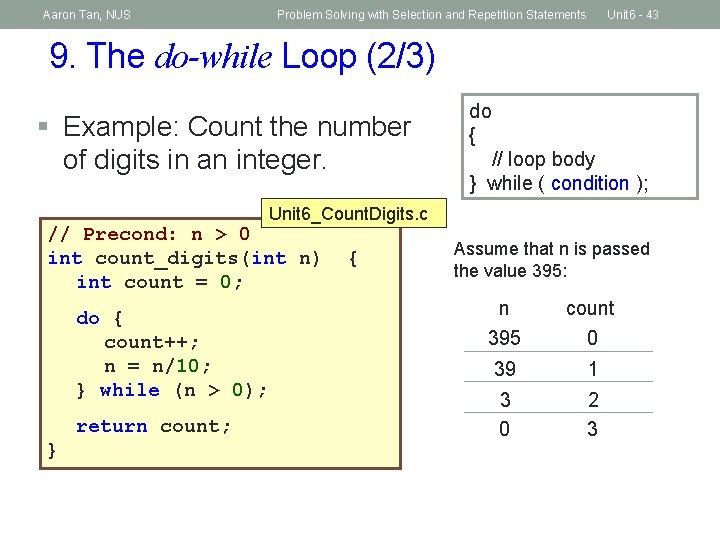Aaron Tan, NUS Problem Solving with Selection and Repetition Statements Unit 6 - 43 9. The do-while Loop (2/3) § Example: Count the number of digits in an integer. do { // loop body } while ( condition ); Unit 6_Count. Digits. c // Precond: n > 0 int count_digits(int n) int count = 0; do { count++; n = n/10; } while (n > 0); return count; } { Assume that n is passed the value 395: n count 395 0 39 1 3 0 2 3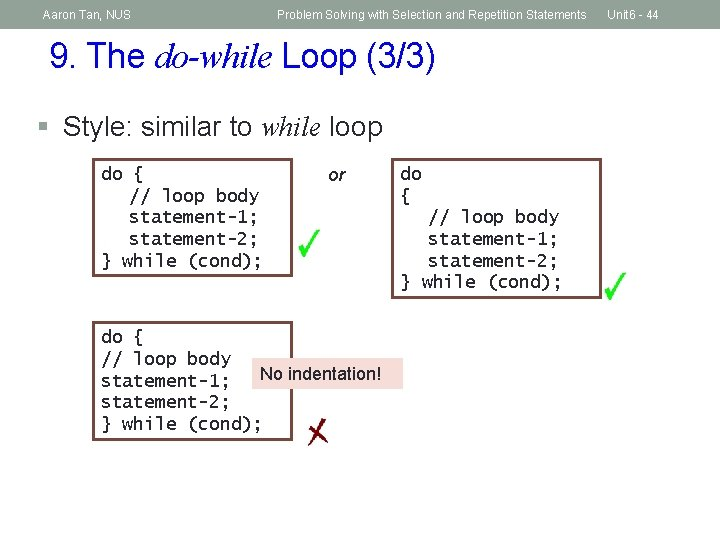Aaron Tan, NUS Problem Solving with Selection and Repetition Statements 9. The do-while Loop (3/3) § Style: similar to while loop do { // loop body statement-1; statement-2; } while (cond); or do { // loop body No indentation! statement-1; statement-2; } while (cond); do { // loop body statement-1; statement-2; } while (cond); Unit 6 - 44Aaron Tan, NUS Problem Solving with Selection and Repetition Statements Unit 6 - 45 9. The do-while Loop: Exercise It’s time to practise Computational Thinking again! § Add the digits in a positive integer. § Eg: 395 17 // Precond: n > 0 int count_digits(int n) int count = 0; do { count++; n = n/10; } while (n > 0); Which concept in Computational Thinking is employed here? { // Precond: n > 0 int add_digits(int n) int sum = 0; do { sum = sum + n%10; n = n/10; } while (n > 0); return count; } return sum; } {Aaron Tan, NUS Problem Solving with Selection and Repetition Statements Unit 6 - 46 10. The for Loop (1/2) for ( initialization; condition; update ) { // loop body } Initialization: initialize the Condition: repeat loop variable while the condition on loop variable is true Update: change value of loop variable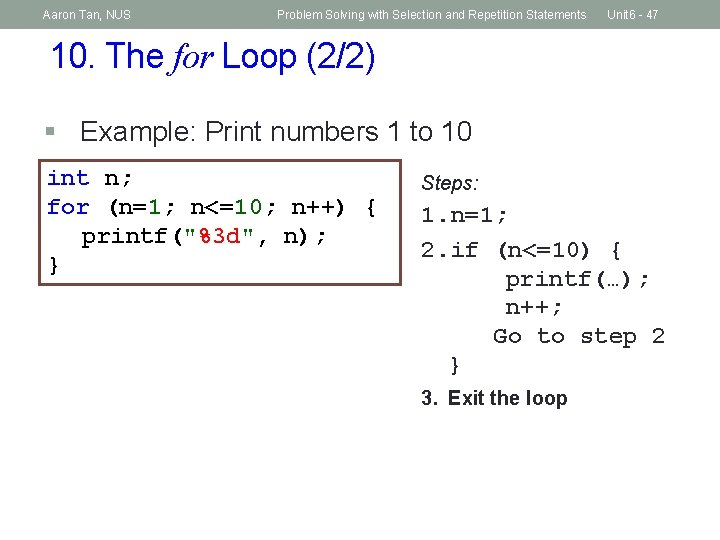Aaron Tan, NUS Problem Solving with Selection and Repetition Statements Unit 6 - 47 10. The for Loop (2/2) § Example: Print numbers 1 to 10 int n; for (n=1; n<=10; n++) { printf("%3 d", n); } Steps: 1. n=1; 2. if (n<=10) { printf(…); n++; Go to step 2 } 3. Exit the loop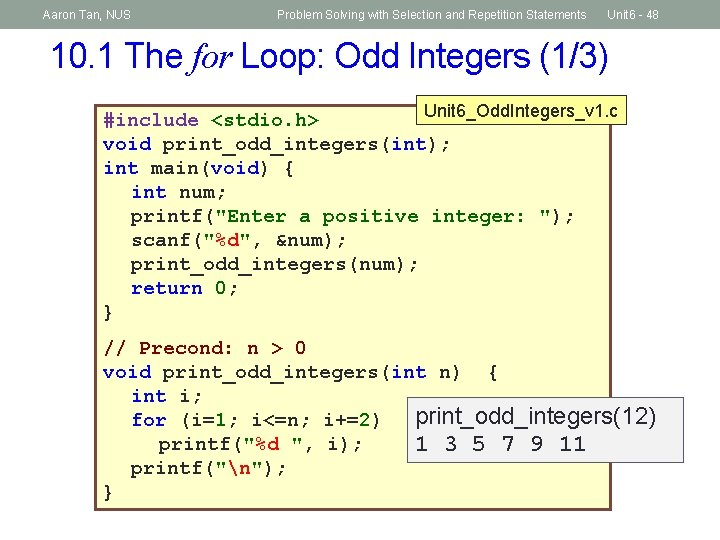Aaron Tan, NUS Problem Solving with Selection and Repetition Statements Unit 6 - 48 10. 1 The for Loop: Odd Integers (1/3) Unit 6_Odd. Integers_v 1. c #include <stdio. h> void print_odd_integers(int); int main(void) { int num; printf("Enter a positive integer: "); scanf("%d", &num); print_odd_integers(num); return 0; } // Precond: n > 0 void print_odd_integers(int n) { int i; print_odd_integers(12) for (i=1; i<=n; i+=2) printf("%d ", i); 1 3 5 7 9 11 printf("n"); }Aaron Tan, NUS Problem Solving with Selection and Repetition Statements Unit 6 - 49 10. 1 The for Loop: Odd Integers (2/3) Unit 6_Odd. Integers_v 2. c // Precond: n > 0 void print_odd_integers(int n) { int i; for (i=1; i<=n; i++) print_odd_integers(12) if (i%2 != 0) 1 3 5 7 9 11 printf("%d ", i); printf("n"); } Unit 6_Odd. Integers_v 3. c // Precond: n > 0 void print_odd_integers(int n) { for ( ; n > 0; n--) Values printed from if (n%2 != 0) printf("%d ", n); largest to smallest. Empty printf("n"); statement print_odd_integers(12) } 11 9 7 5 3 1Aaron Tan, NUS Problem Solving with Selection and Repetition Statements Unit 6 - 50 10. 1 The for Loop: Odd Integers (3/3) Which is better? Unit 6_Odd. Integers_v 1. c // Precond: n > 0 void print_odd_integers(int n) { int i; for (i=1; i<=n; i+=2) printf("%d ", i); printf("n"); } // Precond: n > 0 Unit 6_Odd. Integers_v 2. c void print_odd_integers(int n) { int i; for (i=1; i<=n; i++) if (i%2 != 0) printf("%d ", i); printf("n"); }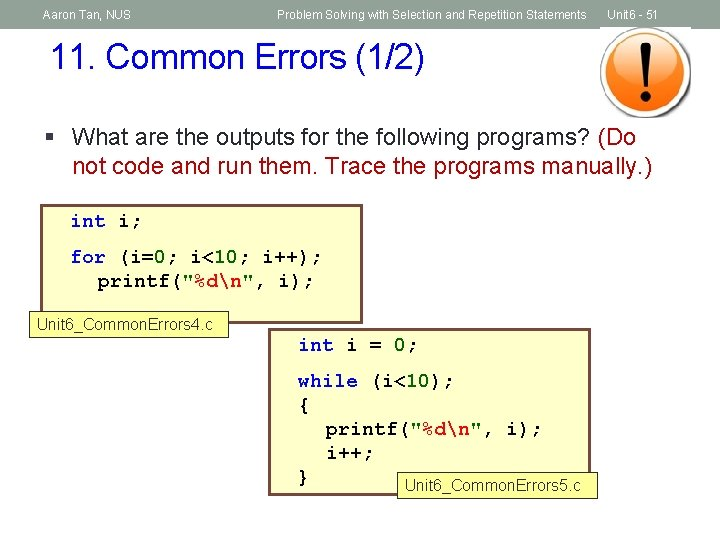Aaron Tan, NUS Problem Solving with Selection and Repetition Statements Unit 6 - 51 11. Common Errors (1/2) § What are the outputs for the following programs? (Do not code and run them. Trace the programs manually. ) int i; for (i=0; i<10; i++); printf("%dn", i); Unit 6_Common. Errors 4. c int i = 0; while (i<10); { printf("%dn", i); i++; } Unit 6_Common. Errors 5. cAaron Tan, NUS Problem Solving with Selection and Repetition Statements Unit 6 - 52 11. Common Errors (2/2) int z = 3; while (z = 1) { printf("z = %dn", z); z = 99; } Unit 6_Common. Errors 6. c § § Off-by-one error; make sure the loop repeats exactly the correct number of iterations. Make sure the loop body contains a statement that will eventually cause the loop to terminate. Common mistake: Using ‘=’ where it should be ‘==’ Common mistake: Putting ‘; ’ where it should not be (just like for the ‘if’ statement)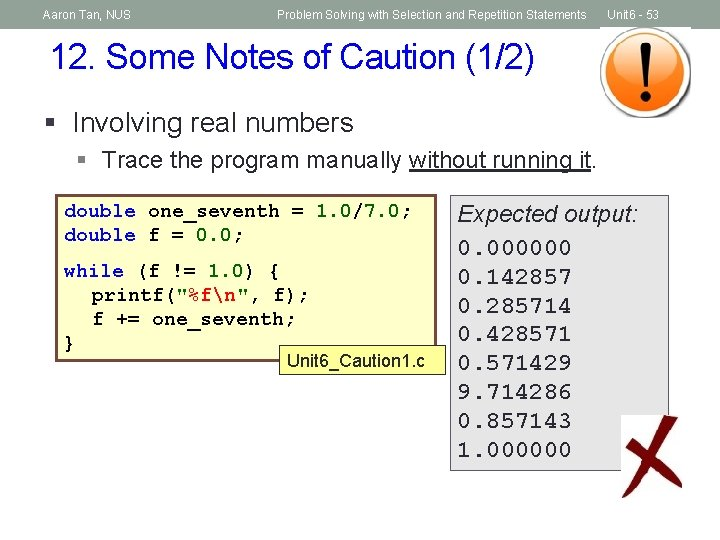Aaron Tan, NUS Problem Solving with Selection and Repetition Statements Unit 6 - 53 12. Some Notes of Caution (1/2) § Involving real numbers § Trace the program manually without running it. double one_seventh = 1. 0/7. 0; double f = 0. 0; while (f != 1. 0) { printf("%fn", f); f += one_seventh; } Unit 6_Caution 1. c Expected output: 0. 000000 0. 142857 0. 285714 0. 428571 0. 571429 9. 714286 0. 857143 1. 000000Aaron Tan, NUS Problem Solving with Selection and Repetition Statements Unit 6 - 54 12. Some Notes of Caution (2/2) § Involving ‘wrap-around’ § Trace the program manually without running it. int a = 2147483646; int i; for (i=1; i<=5; i++) { printf("%dn", a); a++; } Unit 6_Caution 2. c Expected output: 2147483646 2147483647 2147483648 2147483649 2147483650Aaron Tan, NUS Problem Solving with Selection and Repetition Statements Unit 6 - 55 13. Using break in Loop (1/3) § break is used in switch statement § It can also be used in a loop Unit 6_Break. In. Loop. c // without 'break' printf ("Without 'break': n"); for (i=1; i<=5; i++) { printf("%dn", i); printf("Yan"); } // with 'break' printf ("With 'break': n"); for (i=1; i<=5; i++) { printf("%dn", i); if (i==3) break; printf("Yan"); } Without 'break': 1 Ya 2 Ya 3 Ya 4 Ya 5 Ya With 'break': 1 Ya 2 Ya 3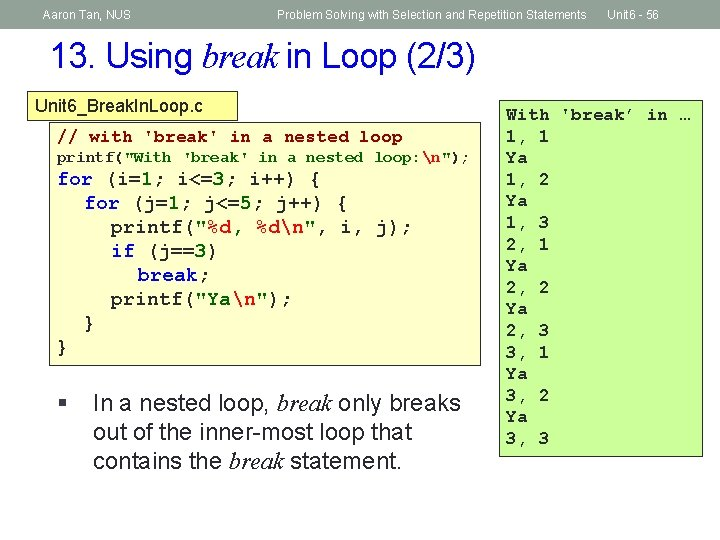Aaron Tan, NUS Problem Solving with Selection and Repetition Statements Unit 6 - 56 13. Using break in Loop (2/3) Unit 6_Break. In. Loop. c // with 'break' in a nested loop printf("With 'break' in a nested loop: n"); for (i=1; i<=3; i++) { for (j=1; j<=5; j++) { printf("%d, %dn", i, j); if (j==3) break; printf("Yan"); } } § In a nested loop, break only breaks out of the inner-most loop that contains the break statement. With 'break’ in … 1, 1 Ya 1, 2 Ya 1, 3 2, 1 Ya 2, 2 Ya 2, 3 3, 1 Ya 3, 2 Ya 3, 3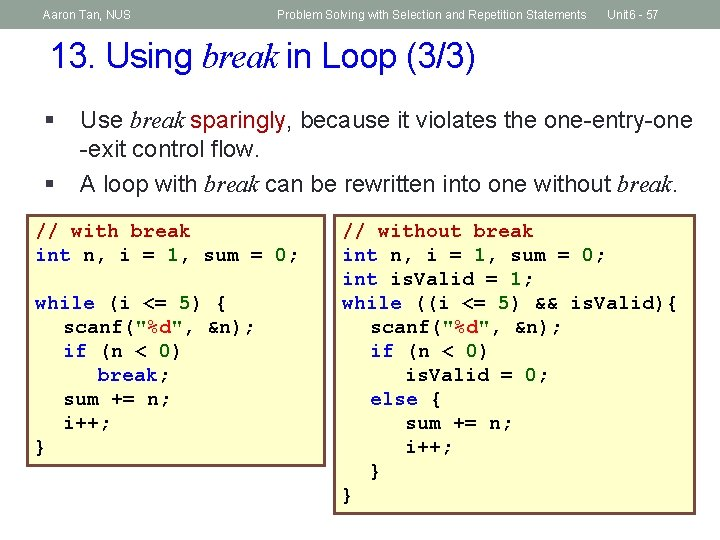Aaron Tan, NUS Problem Solving with Selection and Repetition Statements Unit 6 - 57 13. Using break in Loop (3/3) § § Use break sparingly, because it violates the one-entry-one -exit control flow. A loop with break can be rewritten into one without break. // with break int n, i = 1, sum = 0; while (i <= 5) { scanf("%d", &n); if (n < 0) break; sum += n; i++; } // without break int n, i = 1, sum = 0; int is. Valid = 1; while ((i <= 5) && is. Valid){ scanf("%d", &n); if (n < 0) is. Valid = 0; else { sum += n; i++; } }Aaron Tan, NUS Problem Solving with Selection and Repetition Statements Unit 6 - 58 14. Using continue in Loop (1/2)Without 'continue': § § 1 continue is used even less often than break Ya Test out Unit 6_Continue. In. Loop. c 2 // without 'continue' printf ("Without 'continue': n"); for (i=1; i<=5; i++) { printf("%dn", i); printf("Yan"); } // with 'continue' printf ("With 'continue': n"); for (i=1; i<=5; i++) { printf("%dn", i); if (i==3) The rest of the loop continue; printf("Yan"); body is skipped if (i==3), and it continues } to the next iteration. Ya 3 Ya 4 Ya 5 Ya With 'continue': 1 Ya 2 Ya 3 4 Ya 5 Ya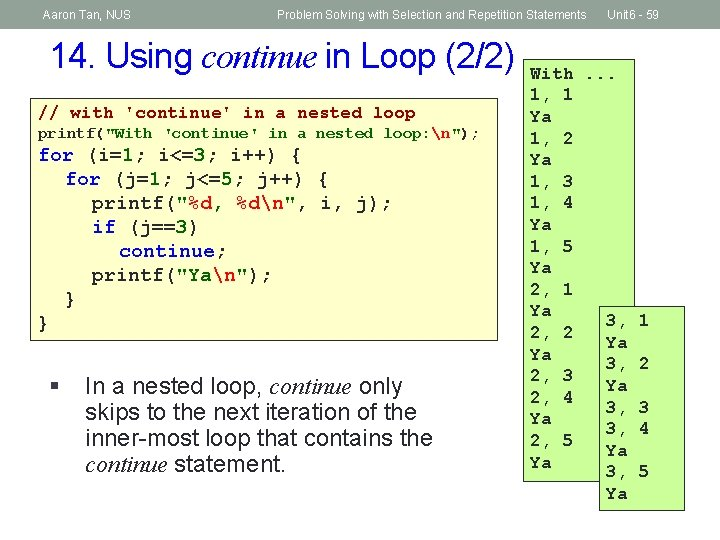Aaron Tan, NUS Problem Solving with Selection and Repetition Statements 14. Using continue in Loop (2/2) // with 'continue' in a nested loop printf("With 'continue' in a nested loop: n"); for (i=1; i<=3; i++) { for (j=1; j<=5; j++) { printf("%d, %dn", i, j); if (j==3) continue; printf("Yan"); } } § In a nested loop, continue only skips to the next iteration of the inner-most loop that contains the continue statement. Unit 6 - 59 With. . . 1, 1 Ya 1, 2 Ya 1, 3 1, 4 Ya 1, 5 Ya 2, 1 Ya 3, 2, 2 Ya Ya 3, 2, 3 Ya 2, 4 3, Ya 3, 2, 5 Ya Ya 3, Ya 1 2 3 4 5Aaron Tan, NUS Problem Solving with Selection and Repetition Statements Unit 6 - 60 Summary n In this unit, you have learned about n The use of if-else construct and switch construct to alter program flow (selection statements) n The use of relational and logical operators in the condition n The use of while, do-while and for loop constructs to repeat a segment of code (repetition statements) n The use of break and continue in a loop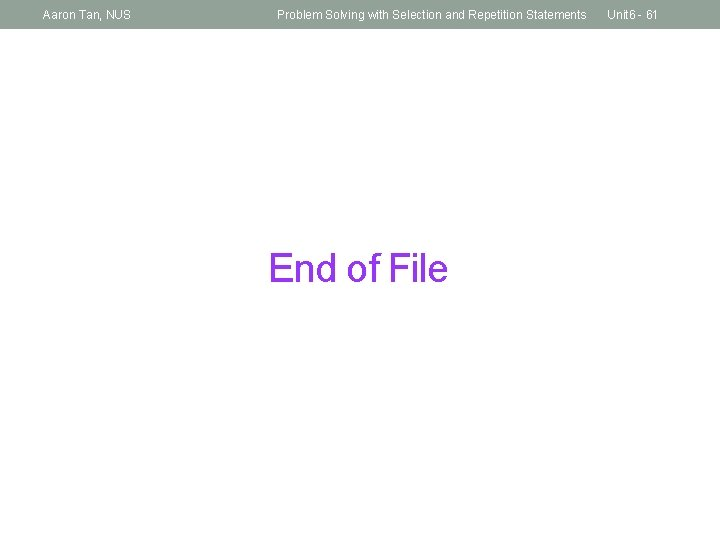Aaron Tan, NUS Problem Solving with Selection and Repetition Statements End of File Unit 6 - 61